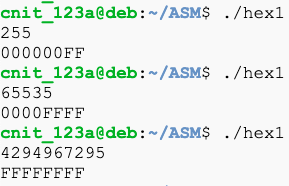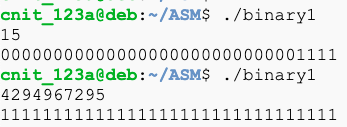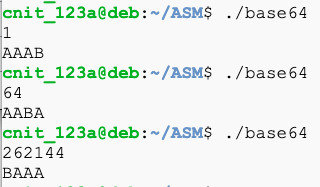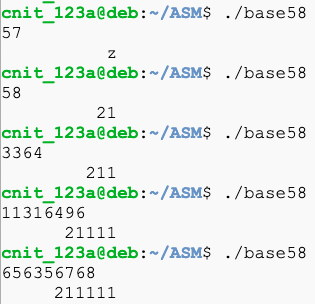ASM 104: Bases & Printing (40 pts)

What You Need

• A Google Cloud Debian 10 Linux Server

Purpose

To practice using the oldest and simplest debugging technique: print statements.

Often you also need to use a real debugger like gdb, which is in a different challenge.

Create this "triple1.asm" file:
section  .text
global   _start

_start:

mov  edx, len_buf_in
mov  ecx, buf_in
mov  ebx, 1
mov  eax, 3             ; 3 = sys_read
int  0x80

mov  al, [buf_in]
shl  al, 1              ; double
mov  [buf_out], al

mov  edx, len_buf_out
mov  ecx, buf_out
mov  ebx, 1
mov  eax, 4             ; 4 = sys_write
int  0x80

mov  eax, 1             ; 1 = exit
int  0x80

section  .data

buf_in       db   "    ", 10, 13
len_buf_in   equ  \$ - buf_in
buf_out      db   "    ", 10, 13
len_buf_out  equ  \$ - buf_out
Compile and run the progam, as shown below.

The goal was to read numbers and triple them, but it fails, as shown below.Create this "triple2.asm" file:
section  .text
global   _start

_start:

mov  edx, len_buf_in
mov  ecx, buf_in
mov  ebx, 1
mov  eax, 3             ; 3 = sys_read
int  0x80

mov  al, [buf_in]
call print_c            ; diagnostic
shl  al, 1              ; double
call print_c            ; diagnostic
call print_c            ; diagnostic
mov  [buf_out], al

mov  edx, len_buf_out
mov  ecx, buf_out
mov  ebx, 1
mov  eax, 4             ; 4 = sys_write
int  0x80

mov  eax, 1             ; 1 = exit
int  0x80

print_c:                    ; prints the character in al
push eax
mov  [print_cs+5], al
mov  edx, len_print_cs
mov  ecx, print_cs
mov  ebx, 1
mov  eax, 4
int  0x80
pop  eax
ret

section  .data

buf_in       db   "    ", 10, 13
len_buf_in   equ  \$ - buf_in
buf_out      db   "    ", 10, 13
len_buf_out  equ  \$ - buf_out

print_cs   db   " D:     ", 10, 13
len_print_cs  equ  \$ - print_cs
Compile and run the progam, as shown below.

Now it prints out the result after each step, as shown below. This makes it easier to find errors.ASM 104.1: Hex (10 pts)

Create a program that accepts a decimal number and converts it to hex, as shown below.Convert this number to hex to obtain the flag:

2880298733

ASM 104.2: Binary (10 pts)

Create a program that accepts a decimal number and converts it to binary, as shown below.Convert this number to binary to obtain the flag:

2865539805

ASM 104.3: Base64 (10 pts)

Create a program that accepts a decimal number and converts it to Base64, as shown below.Convert this number to Base64 to obtain the flag:

803075

ASM 104.4: Base58 (10 pts)

Create a program that accepts a decimal number and converts it to Base58Check, as shown below.Convert this number to Base58 to obtain the flag:

807319263

Sources

System calls in the Linux kernel. Part 1.
X86 Assembly/Interfacing with Linux
Linux System Call Table (32-bit)
List of Linux/i386 system calls
Assembly Programming Tutorial

Posted 7-19-2020